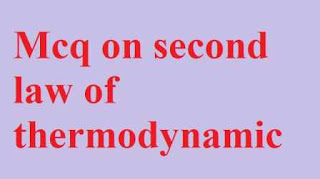### Mcq on second law of thermodynamics (objective questions and answers)

In mechanical engineering mcq website today we solving thermodyanamics subject multiple choice questions Mcq on second law of thermodynamics (objective questions and answers)

Guys this second law of thermodynamics  in thermodynamics mcq with answer is important for all competitive exam so without wasting time start Mcq on 2nd law of thermodynamics .

# Mcq on second law of thermodynamics (objective questions and answers)Mcq on second law of thermodynamics (objective questions and answers)

Second law of thermodynamic Mcq - 1
Which thermodynamics law defined the concept of entropy ?

A. First law of thermodynamics
B. Second law of thermodynamics
C. Third law of thermodynamics
D. Zeroth law of thermodynamics

Second law of thermodynamic Mcq - 2
The law of Second law of thermodynamics is discovered by ........

A. Joule
B. Kelvin
C. Plabt
D. Both B and C

Second law of thermodynamic Mcq - 3
According to 2nd law thermodynamics pmm 2 is ............

A. Possible
B. Impossible
C. Both A and B
D. None of these

Second law of thermodynamic Mcq - 4
The si unit moment of inertia is .........

A. Meter
B. Meter²
C. Meter³
D. Meter⁴

Second law of thermodynamic Mcq - 5
Second law of thermodynamics deals with

A. Conservation of heat
B. Conservation of mass
C. Conservation of energy
D. Conversion of heat into work.

Second law of thermodynamic Mcq - 6
Degress of disorder of a system is called as

A. Entropy
B. Enthalpy
C. Isobaric
D. Internal energy

Second law of thermodynamic Mcq - 7
Si Unit of radius of gyration is .......

A. meter
B. Second
C. meter ²
D. Newton

Second law of thermodynamic Mcq - 8
Pmm 2 machine is Reversible machine of second law of thermodynamics.

A. True
B. False

Second law of thermodynamic Mcq - 9
According to 2nd law thermodynamics heat always flow from

A. Low temperature to high temperature
B. High temperature to low temperature
C. Same temperature
D. None of these

Second law of thermodynamic Mcq - 10
Entropy is defined by second law of thermodynamics.

A. True
B. False

Second law of thermodynamic Mcq - 11
Heat is always flow from high temperature to low temperature

A. True
B. False

Second law of thermodynamic Mcq - 12
It is impossible to convert all heat energy into work energy without any external sources.

A. True
B. False

Second law of thermodynamic Mcq - 13
Heat taken from sink is based on a .........

A. First law of thermodynamics
B. Second law of thermodynamics
C. Third law of thermodynamics
D. Zeroth law of thermodynamics

Second law of thermodynamic Mcq - 14
Second law of thermodynamics defined which thermodynamics properties  ?

A. Entropy
B. Enthalpy
C. Isobaric
D. Internal energy

Second law of thermodynamic Mcq - 15
Heat is flow from high temperature to low temperature this law is defined by ........

A. Joule
B. Kelvin
C. Clausis
D. Amanda's

Second law of thermodynamic Mcq - 16
Refrigeration system work on which law of thermodynamics ?

A. Zeroth law of thermodynamics
B. First law of thermodynamics
C. Second law of thermodynamics
D. Third law of thermodynamics

Second law of thermodynamic Mcq - 17
Pmm 2 machine is impossible machine according to second law of thermodynamics

A. True
B. False

Second law of thermodynamic Mcq - 18
It is impossible to convert all heat energy into work energy without any external sources.

A. Joule
B. Kelvin
C. Clausis
D. Amanda's

Second law of thermodynamic Mcq - 19
According to second law thermodynamics ............. is impossible.

A. Pmm 1
B. Pmm 2
C. Pmm 3
D. Pmm 4

Second law of thermodynamic Mcq - 1
W

A. S
B. S
C. G
D. N